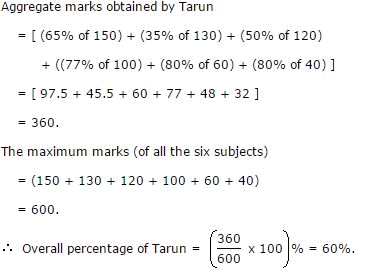# Target SBI PO Mains- Day 13 – Aptitude – DI Table

Direction (1-6 ): Number of Candidates Appeared and Qualified in a Competitive

Examination from Different States over the Years. Number of Candidates Appeared and

Qualified in a Competitive Examination from different States over the Year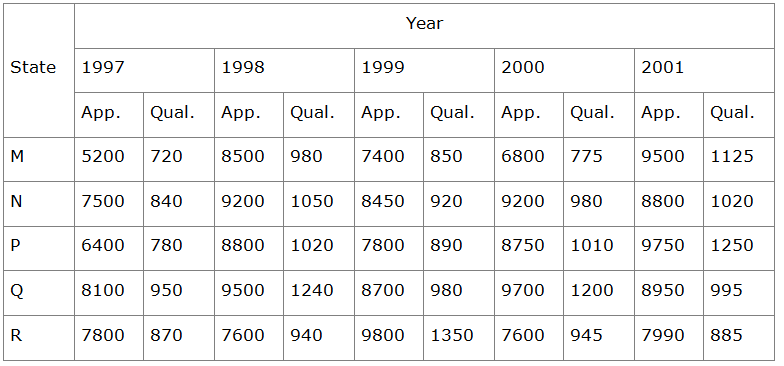1. Total number of candidates qualified from all the states together in 1997 is approximately what percentage of the total number of candidates qualified from all the states together in 1998?

(a) 72%

(b) 77%

(c) 80%

(d) 83%

(e) None of these

(c) 80%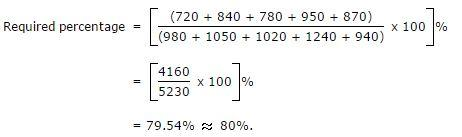2. What is the average candidates who appeared from State Q during the given years?

(a) 8700

(b) 8760

(c) 8990

(d) 8920

(e) None of these

(c) 8990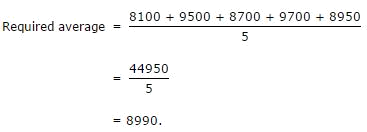3. In which of the given years the number of candidates appeared from State P has maximum percentage of qualified candidates?

(a) 1997

(b) 1998

(c) 1999

(d) 2001

(e) None of these

(d) 2001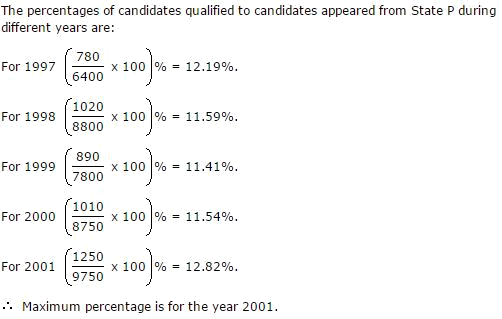4. What is the percentage of candidates qualified from State N for all the years together, over the candidates appeared from State N during all the years together?

(a) 12.36%

(b) 12.16%

(c) 11.47%

(d) 11.15%

(e) None of these

(d) 11.15%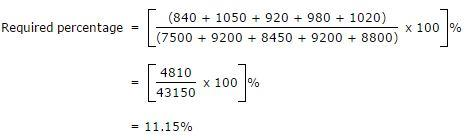5. The percentage of total number of qualified candidates to the total number of appeared candidates among all the five states in 1999 is?

(a) 11.49 %

(b) 11.84 %

(c) 12.21 %

(d) 12.57 %

(e) None of these

Spoiler title
(b) 11.84 %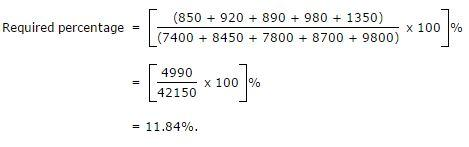6. Combining the states P and Q together in 1998, what is the percentage of the candidates qualified to that of the candidate appeared?

(a) 10.87%

(b) 11.49%

(c) 12.35%

(d) 12.54%

(e) None of these

(c) 12.35%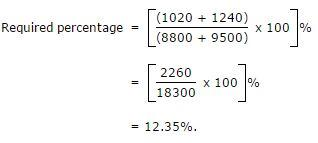Direction (6-10): The following table gives the percentage of marks obtained by seven students in six different subjects in an examination. The Numbers in the Brackets give the Maximum Marks in Each Subject

 Student Subject (Max. Marks) Maths Che Phy Geo His C.S 150 130 120 100 60 40 Ayush 90 50 90 60 70 80 Aman 100 80 80 40 80 70 Sajal 90 60 70 70 90 70 Rohit 80 65 80 80 60 60 Muskan 80 65 85 95 50 90 Tanvi 70 75 65 85 40 60 Tarun 65 35 50 77 80 80

6. What are the average marks obtained by all the seven students in Physics? (rounded off to two digit after decimal)

(a) 77.26

(b) 89.14

(c) 91.37

(d) 96.11

(e) None of these

(b) 89.14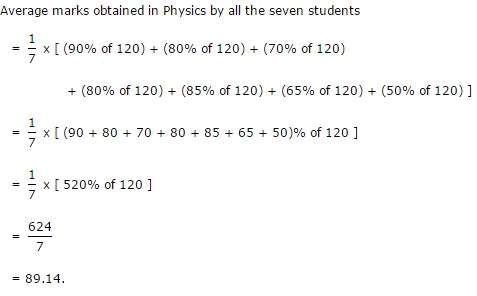7. The number of students who obtained 60% and above marks in all subjects is?

(a) 1

(b) 2

(c) 3

(d) Cannot be determined

(e) None of these

(b) From the table it is clear that Sajal and Rohit have 60% or more marks in each of the six subjects.

8. (d) Aggregate marks obtained by Sajal

= [ (90% of 150) + (60% of 130) + (70% of 120)

+ (70% of 100) + (90% of 60) + (70% of 40) ]

= [ 135 + 78 + 84 + 70 + 54 + 28 ]

= 449.

8. What was the aggregate of marks obtained by Sajal in all the six subjects?

(a) 409

(b) 419

(c) 429

(d) 449

(e) None of these

(d) Aggregate marks obtained by Sajal

= [ (90% of 150) + (60% of 130) + (70% of 120)

+ (70% of 100) + (90% of 60) + (70% of 40) ]

= [ 135 + 78 + 84 + 70 + 54 + 28 ]

= 449.

9. In which subject is the overall percentage the best?

(a) Maths

(b) Chemistry

(c) Physics

(d) History

(e) None of these

a) We shall find the overall percentage (for all the seven students) with respect to each subject.

The overall percentage for any subject is equal to the average of percentages obtained by all the seven students since the maximum marks for any subject is the same for all the students.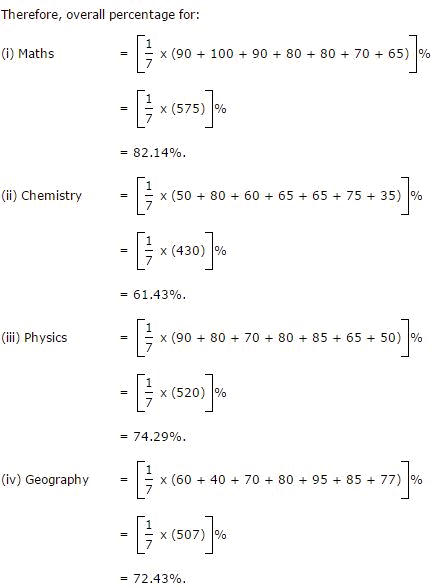10. What is the overall percentage of Tarun?

(a) 52.5%

(b) 55%

(c) 60%

(d) 63%

(e) None of these